# Existence result for semilinear elliptic systems involving critical exponents

## Abstract

In this paper we deal with the existence of a positive solution for a class of semilinear systems of multi-singular elliptic equations which involve Sobolev critical exponents. In fact, by the analytic techniques and variational methods, we prove that there exists at least one positive solution for the system.

MSC: 35J60, 35B33.

## 1 Introduction

We consider the following elliptic system:

$\left\{\begin{array}{cc}Lu=\frac{\sigma \alpha }{{2}^{\ast }}u|u{|}^{\alpha -2}|\nu {|}^{\beta }+\eta u|u{|}^{{2}^{\ast }-2}+{a}_{1}u+{a}_{2}\nu ,\hfill & \phantom{\rule{1em}{0ex}}\mathrm{\Omega },\hfill \\ L\nu =\frac{\sigma \beta }{{2}^{\ast }}\nu |\nu {|}^{\beta -2}|u{|}^{\alpha }+\lambda \nu |\nu {|}^{{2}^{\ast }-2}+{a}_{2}u+{a}_{3}\nu ,\hfill & \phantom{\rule{1em}{0ex}}\mathrm{\Omega },\hfill \\ u=\nu =0,\hfill & \phantom{\rule{1em}{0ex}}\partial \mathrm{\Omega },\hfill \end{array}$
(1.1)

where $\mathrm{\Omega }\subset {\mathbb{R}}^{N}$ ($N\ge 3$) is a smooth bounded domain such that ${\xi }_{i}\in \mathrm{\Omega }$, $i=1,2,\dots ,k$, $k\ge 2$, are different points, $0\le {\mu }_{i}<\overline{\mu }:={\left(\frac{N-2}{2}\right)}^{2}$, $L:=-\mathrm{\Delta }\cdot -{\sum }_{i=1}^{k}{\mu }_{i}\frac{\cdot }{|x-{\xi }_{i}{|}^{2}}$, $\eta ,\lambda ,\sigma \ge 0$, ${a}_{1},{a}_{2},{a}_{3}\in \mathbb{R}$, $1<\alpha$, $\beta <{2}^{\ast }-1$, $\alpha +\beta ={2}^{\ast }$.

We work in the product space $H×H$, where the space $H:={H}_{0}^{1}\left(\mathrm{\Omega }\right)$ is the completion of ${C}_{0}^{\mathrm{\infty }}\left(\mathrm{\Omega }\right)$ with respect to the norm ${\left({\int }_{\mathrm{\Omega }}|\mathrm{\nabla }\cdot {|}^{2}\phantom{\rule{0.2em}{0ex}}dx\right)}^{\frac{1}{2}}$.

In resent years many publications  concerning semilinear elliptic equations involving singular points and the critical Sobolev exponent have appeared. Particularly in the last decade or so, many authors used the variational method and analytic techniques to study the existence of positive solutions of systems of the form of (1.1) or its variations; see, for example, .

Before stating the main result, we clarify some terminology. Since our method is variational in nature, we need to define the energy functional of (1.1) on $H×H$

$\begin{array}{rcl}J\left(u,\nu \right)& =& \frac{1}{2}{\int }_{\mathrm{\Omega }}\left(|\mathrm{\nabla }u{|}^{2}+|\mathrm{\nabla }\nu {|}^{2}-\sum _{i=1}^{k}\frac{{\mu }_{i}\left({u}^{2}+{\nu }^{2}\right)}{|x-{\xi }_{i}{|}^{2}}\right)\phantom{\rule{0.2em}{0ex}}dx\\ -\frac{1}{2}{\int }_{\mathrm{\Omega }}\left({a}_{1}{u}^{2}+2{a}_{2}u\nu +{a}_{3}{\nu }^{2}\right)\phantom{\rule{0.2em}{0ex}}dx-\sigma \frac{\sigma }{{2}^{\ast }}{\int }_{\mathrm{\Omega }}|u{|}^{\alpha }|u{|}^{\beta }\phantom{\rule{0.2em}{0ex}}dx\\ -\frac{\eta }{{2}^{\ast }}{\int }_{\mathrm{\Omega }}|u{|}^{{2}^{\ast }}\phantom{\rule{0.2em}{0ex}}dx-\frac{\lambda }{{2}^{\ast }}{\int }_{\mathrm{\Omega }}|\nu {|}^{{2}^{\ast }}\phantom{\rule{0.2em}{0ex}}dx.\end{array}$

Then $J\left(u,\nu \right)$ belongs to ${C}^{1}\left(H×H,\mathbb{R}\right)$. A pair of functions $\left({u}_{0},{\nu }_{0}\right)\in H×H$ is said to be a solution of (1.1) if $\left({u}_{0},{\nu }_{0}\right)\ne \left(0,0\right)$, and for all $\left(\phi ,\varphi \right)\in H×H$, we have

$\begin{array}{c}{\int }_{\mathrm{\Omega }}\left(\mathrm{\nabla }{u}_{0}\cdot \mathrm{\nabla }\phi +\mathrm{\nabla }{\nu }_{0}\cdot \mathrm{\nabla }\varphi -\sum _{i=1}^{k}\frac{{\mu }_{i}\left({u}_{0}\phi +{\nu }_{0}\varphi \right)}{|x-{\xi }_{i}{|}^{2}}-\left({a}_{1}{u}_{0}\phi +{a}_{2}\phi {\nu }_{0}+{a}_{2}\varphi {u}_{0}+{a}_{3}{\nu }_{0}\varphi \right)\hfill \\ \phantom{\rule{1em}{0ex}}-\sigma \left(\alpha \phi {u}_{0}|{u}_{0}{|}^{\alpha -2}|{\nu }_{0}{|}^{\beta }+\beta \varphi {\nu }_{0}|{\nu }_{0}{|}^{\beta -2}|{u}_{0}{|}^{\alpha }\right)\hfill \\ \phantom{\rule{1em}{0ex}}-\eta |{u}_{0}{|}^{{2}^{\ast }-2}{u}_{0}\phi -\lambda |{\nu }_{0}{|}^{{2}^{\ast }-2}{\nu }_{0}\varphi \right)\phantom{\rule{0.2em}{0ex}}dx=〈{J}^{\prime }\left({u}_{0},{\nu }_{0}\right),\left(\phi ,\varphi \right)〉=0.\hfill \end{array}$

Standard elliptic arguments show that

$u,\nu \in {C}^{2}\left(\mathrm{\Omega }\mathrm{\setminus }\left\{{\xi }_{1},\dots ,{\xi }_{k}\right\}\right)\cap {C}^{1}\left(\overline{\mathrm{\Omega }}\mathrm{\setminus }\left\{{\xi }_{1},\dots ,{\xi }_{k}\right\}\right).$

The following assumptions are needed:

(${\mathcal{H}}_{1}$) $\eta +\lambda +\sigma >0$, $0\le {\mu }_{1}\le {\mu }_{2}\le \cdots \le {\mu }_{k}<\overline{\mu }-1$ and ${\sum }_{i=1}^{k}{\mu }_{i}<\overline{\mu }$, $\alpha +\beta >1$, $\alpha +\beta ={2}^{\ast }$, ${a}_{1},{a}_{2},{a}_{3}>0$, ${a}_{1}{a}_{3}-{a}_{2}^{2}>0$,

(${\mathcal{H}}_{2}$) $0<{\lambda }_{1}\le {\lambda }_{2}<{\mathrm{\Lambda }}_{1}\left(\mu \right)$, where ${\mathrm{\Lambda }}_{1}\left(\mu \right)$ is the first eigenvalue of L, ${\lambda }_{1}$, ${\lambda }_{2}$ are the eigenvalues of the matrix $A=\left(\begin{array}{cc}{a}_{1}& {a}_{2}\\ {a}_{2}& {a}_{3}\end{array}\right)$.

The quadratic from $Q\left(u,\nu \right):=\left(u,\nu \right)A{\left(u,\nu \right)}^{T}={a}_{1}{u}^{2}+2{a}_{2}u\nu +{a}_{3}{\nu }^{2}$ is positively defined and satisfies

${\lambda }_{1}\left({u}^{2}+{\nu }^{2}\right)\le {a}_{1}{u}^{2}+2{a}_{2}u\nu +{a}_{3}{\nu }^{2}\le {\lambda }_{2}\left({u}^{2}+{\nu }^{2}\right)\phantom{\rule{1em}{0ex}}\mathrm{\forall }u,\nu \in H.$
(1.2)

Our main results are as follows.

Theorem 1.1 Suppose (${\mathcal{H}}_{1}$) holds. Then for any solution $\left(u,v\right)\in H×H$ of problem (1.1), there exists a positive constant ${C}_{1}$ such that

$max\left\{|u\left(x\right)|,|\nu \left(x\right)|\right\}\le {C}_{1}|x-{\xi }_{i}{|}^{-\left(\sqrt{\overline{\mu }}-\sqrt{\overline{\mu }-{\mu }_{i}}\right)},\phantom{\rule{1em}{0ex}}\mathrm{\forall }x\in {B}_{{\rho }_{1}}\left({\xi }_{i}\right)\mathrm{\setminus }\left\{{\xi }_{i}\right\},$

where ${\rho }_{1}>0$ and ${B}_{{\rho }_{1}}\left({\xi }_{i}\right)\subset \mathrm{\Omega }$.

Theorem 1.2 Suppose (${\mathcal{H}}_{1}$) holds. Then for any positive solution $\left(u,v\right)\in H×H$ of problem (1.1), there exists a positive constant ${C}_{2}$ such that ${B}_{{\rho }_{2}}\left({\xi }_{i}\right)\subset \mathrm{\Omega }$ and

$min\left\{u\left(x\right),\nu \left(x\right)\right\}\ge {C}_{2}|x-{\xi }_{i}{|}^{-\left(\sqrt{\overline{\mu }}-\sqrt{\overline{\mu }-{\mu }_{i}}\right)},\phantom{\rule{1em}{0ex}}\mathrm{\forall }x\in {B}_{{\rho }_{2}}\left({\xi }_{i}\right)\mathrm{\setminus }\left\{{\xi }_{i}\right\},$

where ${\rho }_{2}>0$.

Theorem 1.3 Suppose (${\mathcal{H}}_{1}$), (${\mathcal{H}}_{2}$) hold. Then the problem (1.1) has a positive solution.

## 2 Preliminaries

On $H×H$, we use the norm

${\parallel \left(u,\nu \right)\parallel }_{H×H}={\left({\int }_{\mathrm{\Omega }}\left(|\mathrm{\nabla }u{|}^{2}+|\mathrm{\nabla }\nu {|}^{2}\right)\phantom{\rule{0.2em}{0ex}}dx\right)}^{\frac{1}{2}}.$

Using the Young inequality, the following best constant is well defined:

${S}_{{\mu }_{i}}:=\underset{u\in {D}^{1,2}\left({\mathbb{R}}^{N}\right)\mathrm{\setminus }\left\{0\right\}}{inf}\frac{{\int }_{{\mathbb{R}}^{N}}\left(|\mathrm{\nabla }u{|}^{2}-{\mu }_{i}\frac{{u}^{2}}{|x-{\xi }_{i}{|}^{2}}\right)\phantom{\rule{0.2em}{0ex}}dx}{{\left({\int }_{{\mathbb{R}}^{N}}|u{|}^{{2}^{\ast }}\phantom{\rule{0.2em}{0ex}}dx\right)}^{2/{2}^{\ast }}},$
(2.1)

where ${D}^{1,2}\left({\mathbb{R}}^{N}\right)$ is the completion of ${C}_{0}^{\mathrm{\infty }}\left({\mathbb{R}}^{N}\right)$ with respect to the norm ${\left({\int }_{{\mathbb{R}}^{N}}|\mathrm{\nabla }\cdot {|}^{2}\phantom{\rule{0.2em}{0ex}}dx\right)}^{\frac{1}{2}}$.

We infer that ${S}_{{\mu }_{i}}$ is attained in ${\mathbb{R}}^{N}$ by the functions

${V}_{{\mu }_{i},\epsilon }^{{\xi }_{i}}\left(x\right)={\epsilon }^{\frac{2-N}{2}}{U}_{{\mu }_{i}}\left(\frac{x-{\xi }_{i}}{\epsilon }\right),\phantom{\rule{1em}{0ex}}\mathrm{\forall }\epsilon >0,$

where

${U}_{{\mu }_{i}}\left(x-{\xi }_{i}\right)=\frac{{\left(\frac{2N\left(\overline{\mu }-{\mu }_{i}\right)}{\sqrt{\overline{\mu }}}\right)}^{\sqrt{\overline{\mu }}/2}}{|x-{\xi }_{i}{|}^{\left(\sqrt{\overline{\mu }}-\sqrt{\overline{\mu }-{\mu }_{i}}\right)}{\left(1+|x-{\xi }_{i}{|}^{\frac{2\sqrt{\overline{\mu }-{\mu }_{i}}}{\sqrt{\overline{\mu }}}}\right)}^{\sqrt{\overline{\mu }}}}.$

For all $\eta ,\lambda ,\sigma \ge 0$, $\eta +\lambda +\sigma >0$, $\alpha +\beta >1$, $\alpha +\beta ={2}^{\ast }$, by the Young and Hardy-Sobolev inequalities, the following constant is well defined on $\mathcal{D}:={\left({D}^{1,2}\left({\mathbb{R}}^{N}\right)\mathrm{\setminus }\left\{0\right\}\right)}^{2}$:

${S}_{\eta ,\lambda ,\sigma }\left({\mu }_{i}\right):=\underset{\left(u,\nu \right)\in \mathcal{D}}{inf}\frac{{\int }_{{\mathbb{R}}^{N}}\left(|\mathrm{\nabla }u{|}^{2}+|\mathrm{\nabla }\nu {|}^{2}-{\mu }_{i}\frac{{u}^{2}+{\nu }^{2}}{|x-{\xi }_{i}{|}^{2}}\right)\phantom{\rule{0.2em}{0ex}}dx}{{\left({\int }_{{\mathbb{R}}^{N}}\left(\eta |u{|}^{{2}^{\ast }}+\lambda |\nu {|}^{{2}^{\ast }}+\sigma |u{|}^{\alpha }|\nu {|}^{\beta }\right)\phantom{\rule{0.2em}{0ex}}dx\right)}^{2/{2}^{\ast }}}.$
(2.2)

Set

${u}_{\mu ,\epsilon }^{\xi }\left(x\right)=\psi \left(x\right){V}_{\mu ,\epsilon }^{\xi }\left(x\right)={\epsilon }^{\frac{2-N}{2}}\psi \left(x\right){U}_{\mu }\left(\frac{x-\xi }{\epsilon }\right),$

where $\xi \in \mathrm{\Omega }$, $0\le \mu <\overline{\mu }$, $\psi \in {C}_{0}^{\mathrm{\infty }}\left({B}_{\rho }\left(\xi \right)\right)$ satisfies $0\le \psi \le 1$ and $\psi \equiv 1$, $\mathrm{\forall }x\in {B}_{\frac{\rho }{2}\left(\xi \right)}$, for all $\rho >0$ small. Then for any $0<\mu <\overline{\mu }$, by  we have the following estimates:

$\begin{array}{c}{\int }_{\mathrm{\Omega }}\left(|\mathrm{\nabla }{u}_{\mu ,\epsilon }^{\xi }{|}^{2}-\mu \frac{{\left({u}_{\mu ,\epsilon }^{\xi }\right)}^{2}}{|x-\xi {|}^{2}}\right)\phantom{\rule{0.2em}{0ex}}dx={S}_{\mu }^{\frac{N}{2}}+o\left({\epsilon }^{2\sqrt{\overline{\mu }}-\mu }\right),\hfill \\ {\int }_{\mathrm{\Omega }}|{u}_{\mu ,\epsilon }^{\xi }{|}^{{2}^{\ast }}\phantom{\rule{0.2em}{0ex}}dx={S}_{\mu }^{\frac{N}{2}}+o\left({\epsilon }^{\frac{2N}{N-2}\sqrt{\overline{\mu }}-\mu }\right),\hfill \end{array}$

and for any $a\in {\mathbb{R}}^{N}\mathrm{\setminus }\left\{0\right\}$,

where ${C}_{\mu }={\left(\frac{4N\left(\overline{\mu }-\mu \right)}{N-2}\right)}^{\frac{N-2}{4}}$, ${\omega }_{N}$ is the volume of the unit ball in ${\mathbb{R}}^{N}$.

## 3 Asymptotic behavior of solutions

Proof of Theorem 1.1 Suppose $\left({u}_{0},{\nu }_{0}\right)\in H×H$ is a nontrivial solution to problem (1.1). For all $0\le {\mu }_{i}\le \overline{\mu }$ define

It is not difficult to verify that $u,\nu \in {H}_{0}^{1}\left(\mathrm{\Omega },|x-{\xi }_{i}{|}^{-2\gamma }\right)$ and satisfy

$\left\{\begin{array}{c}-div\left({|x-{\xi }_{i}|}^{-2\gamma }\mathrm{\nabla }u\right)\hfill \\ \phantom{\rule{1em}{0ex}}=\frac{\sigma \alpha }{{2}^{\ast }}{|x-{\xi }_{i}|}^{-{2}^{\ast }\gamma }u|u{|}^{\alpha -2}|\nu {|}^{\beta }+\eta {|x-{\xi }_{i}|}^{-{2}^{\ast }\gamma }u|u{|}^{{2}^{\ast }-2}\hfill \\ \phantom{\rule{2em}{0ex}}+{a}_{1}{|x-{\xi }_{i}|}^{-2\gamma }u+{a}_{2}{|x-{\xi }_{i}|}^{-2\gamma }\nu +{\sum }_{\begin{array}{c}j=1\\ j\ne i\end{array}}^{k}\frac{{\mu }_{j}}{{|x-{\xi }_{j}|}^{2}}{|x-{\xi }_{i}|}^{-2\gamma }u,\hfill \\ -div\left({|x-{\xi }_{i}|}^{-2\gamma }\mathrm{\nabla }\nu \right)\hfill \\ \phantom{\rule{1em}{0ex}}=\frac{\sigma \beta }{{2}^{\ast }}{|x-{\xi }_{i}|}^{-{2}^{\ast }\gamma }\nu |\nu {|}^{\beta -2}|u{|}^{\alpha }+\lambda {|x-{\xi }_{i}|}^{-{2}^{\ast }\gamma }\nu |\nu {|}^{{2}^{\ast }-2}\hfill \\ \phantom{\rule{2em}{0ex}}+{a}_{2}{|x-{\xi }_{i}|}^{-2\gamma }u+{a}_{3}{|x-{\xi }_{i}|}^{-2\gamma }\nu +{\sum }_{\begin{array}{c}j=1\\ j\ne i\end{array}}^{k}\frac{{\mu }_{j}}{{|x-{\xi }_{j}|}^{2}}{|x-{\xi }_{i}|}^{-2\gamma }\nu .\hfill \end{array}$
(3.1)

Let $R>0$ small enough such that ${B}_{R}\left({\xi }_{i}\right)\subset \mathrm{\Omega }$ and ${\xi }_{i}\notin {B}_{R}\left({\xi }_{i}\right)$ for $j\ne i$. Also, let ${\phi }_{i}\in {C}_{0}^{\mathrm{\infty }}\left({B}_{R}\left({\xi }_{i}\right)\right)$ be a cut-off function. Set

${u}_{n}:=min\left\{|u|,n\right\};\phantom{\rule{2em}{0ex}}{\nu }_{n}:=min\left\{|\nu |,n\right\};\phantom{\rule{2em}{0ex}}{\varphi }_{{1}_{i}}:={\phi }_{i}^{2}u{u}_{n}^{2\left(s-1\right)},\phantom{\rule{2em}{0ex}}{\varphi }_{{2}_{i}}:={\phi }_{i}^{2}\nu {\nu }_{n}^{2\left(s-1\right)},$

where $s,n>1$. Multiplying the first equation of (3.1) by ${\varphi }_{{1}_{i}}$ and the second one by ${\varphi }_{{2}_{i}}$ respectively and integrating, we have

$\begin{array}{rcl}{\int }_{\mathrm{\Omega }}{|x-{\xi }_{i}|}^{-2\gamma }\mathrm{\nabla }u\mathrm{\nabla }{\varphi }_{{1}_{i}}& =& \frac{\sigma \alpha }{{2}^{\ast }}{\int }_{\mathrm{\Omega }}{|x-{\xi }_{i}|}^{-{2}^{\ast }\gamma }u|u{|}^{\alpha -2}|\nu {|}^{\beta }{\varphi }_{{1}_{i}}+\eta {\int }_{\mathrm{\Omega }}{|x-{\xi }_{i}|}^{-{2}^{\ast }\gamma }\\ ×u|u{|}^{{2}^{\ast }-2}{\varphi }_{{1}_{i}}+{a}_{1}{\int }_{\mathrm{\Omega }}{|x-{\xi }_{i}|}^{-2\gamma }u{\varphi }_{{1}_{i}}+{a}_{2}{\int }_{\mathrm{\Omega }}{|x-{\xi }_{i}|}^{-2\gamma }\\ ×\nu {\varphi }_{{1}_{i}}+\sum _{\begin{array}{c}j=1\\ j\ne i\end{array}}^{k}\frac{{\mu }_{j}}{{|x-{\xi }_{j}|}^{2}}{|x-{\xi }_{i}|}^{-2\gamma }u{\varphi }_{{1}_{i}}.\end{array}$

Note that $\mathrm{\nabla }{\varphi }_{{1}_{i}}=2{\phi }_{i}u{u}_{n}^{2\left(s-1\right)}\mathrm{\nabla }{\phi }_{i}+{\phi }_{i}^{2}{u}_{n}^{2\left(s-1\right)}\mathrm{\nabla }u+2\left(s-1\right){\phi }_{i}^{2}{u}_{n}^{2\left(s-1\right)}\mathrm{\nabla }{u}_{n}$.

Then

$\begin{array}{rcl}{\int }_{\mathrm{\Omega }}{|x-{\xi }_{i}|}^{-2\gamma }\mathrm{\nabla }u\mathrm{\nabla }{\varphi }_{{1}_{i}}& =& 2{\int }_{\mathrm{\Omega }}{|x-{\xi }_{i}|}^{-2\gamma }{\phi }_{i}u{u}_{n}^{2\left(s-1\right)}\mathrm{\nabla }{\phi }_{i}\mathrm{\nabla }u+{\int }_{\mathrm{\Omega }}{|x-{\xi }_{i}|}^{-2\gamma }{\phi }_{i}^{2}\\ ×{u}_{n}^{2\left(s-1\right)}|\mathrm{\nabla }u{|}^{2}+2\left(s-1\right){\int }_{\mathrm{\Omega }}{|x-{\xi }_{i}|}^{-2\gamma }{\phi }_{i}^{2}{u}_{n}^{2\left(s-1\right)}|\mathrm{\nabla }{u}_{n}{|}^{2}.\end{array}$

By the Cauchy inequality and the Young inequality, we get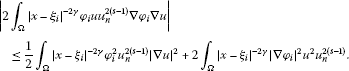(3.2)

The same result holds for ${\int }_{\mathrm{\Omega }}{|x-{\xi }_{i}|}^{-2\gamma }\mathrm{\nabla }{\varphi }_{{2}_{i}}\mathrm{\nabla }\nu$.

By letting ${\psi }_{1}\left(x\right)={\phi }_{i}u{u}_{n}^{\left(s-1\right)}$, ${\psi }_{2}\left(x\right)={\phi }_{i}\nu {\nu }_{n}^{\left(s-1\right)}$, we have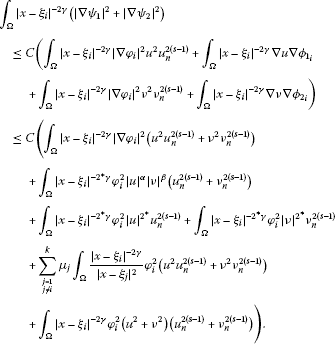(3.3)

Using Caffarelli-Kohn-Nirenberg inequality , we infer that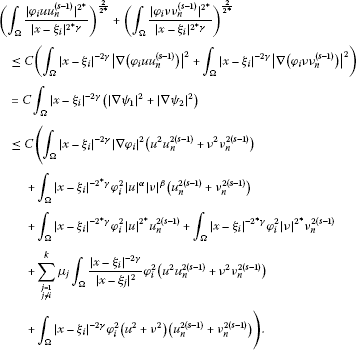(3.4)

Define

$\omega \left(x\right):=max\left\{u\left(x\right),\nu \left(x\right)\right\},\phantom{\rule{2em}{0ex}}{\omega }_{n}\left(x\right):=min\left\{\omega \left(x\right),n\right\}.$

Then ${\omega }_{n}\left(x\right):=max\left\{{u}_{n}\left(x\right),{\nu }_{n}\left(x\right)\right\}$. Now, from the Hölder inequality, we deduce that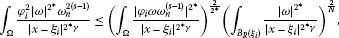(3.5)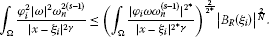(3.6)

In the sequel, we have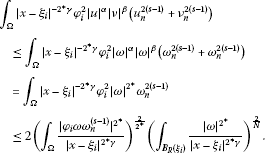(3.7)

By the choice of ${\phi }_{i}$, we obtain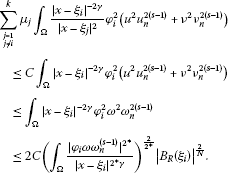(3.8)

So, from (3.4) to (3.8) it follows that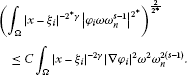(3.9)

Take $s=\frac{{2}^{\ast }}{2}$ and ${\phi }_{i}\left(x\right)$ to be a constant near the zero. Letting $n\to \mathrm{\infty }$, we infer that $\omega \in {L}^{\frac{{2}^{{\ast }^{2}}}{2}}\left({B}_{R}\left({\xi }_{i}\right),{|x-{\xi }_{i}|}^{-{2}^{\ast }\gamma }\right)$ and so

$u,\nu \in {L}^{\frac{{2}^{{\ast }^{2}}}{2}}\left({B}_{R}\left({\xi }_{i}\right),{|x-{\xi }_{i}|}^{-{2}^{\ast }\gamma }\right).$
(3.10)

Suppose $r>0$ is sufficiently small such that $r+l and ${\phi }_{i}$ is a cut-off function with the properties $|\mathrm{\nabla }{\phi }_{i}|<\frac{1}{l}$ and ${\phi }_{i}\left(x\right)=1$ in ${B}_{r}\left({\xi }_{i}\right)$.

Set $t:=\frac{{2}^{{\ast }^{2}}}{2\left({2}^{\ast }-2\right)}$, $\delta :=2\gamma t-{2}^{\ast }\gamma \left(t-1\right)$.

Then we have the following results: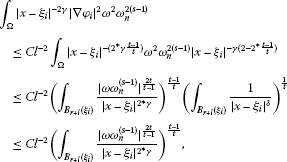(3.11)

where we used the Hölder inequality. From (3.9) in combination with (3.11), it follows that

${\left({\int }_{{B}_{r+l}\left({\xi }_{i}\right)}{|x-{\xi }_{i}|}^{{2}^{\ast }\gamma }|\omega {|}^{{2}^{\ast }s}\right)}^{\frac{1}{{2}^{\ast }s}}\le {C}^{\frac{1}{2s}}{l}^{-\frac{1}{2s}}{\left({\int }_{{B}_{r+l}\left({\xi }_{i}\right)}{|x-{\xi }_{i}|}^{{2}^{\ast }\gamma }|\omega {|}^{{\overline{p}}_{0}s}\right)}^{\frac{1}{{\overline{p}}_{0}s}},$
(3.12)

where ${\overline{p}}_{0}=\frac{2t}{t-1}<{2}^{\ast }$.

Denote $s={\chi }^{j}$, $\chi =\frac{p}{{\overline{p}}_{0}}$ and $l={\rho }^{j}$, $j\ge 1$, where $\chi \ge 1$, ${2}^{\ast }\gamma and ${\overline{p}}_{0}{\chi }^{j}=p{\chi }^{j-1}$. Using (3.12) recursively, we get

$\begin{array}{rcl}{\left({\int }_{{B}_{r}\left({\xi }_{i}\right)}|\omega {|}^{{2}^{\ast }{\chi }^{j}}\right)}^{\frac{1}{{2}^{\ast }{\chi }^{j}}}& \le & {r}^{\frac{\gamma }{{\chi }^{j}}}{\left({\int }_{{B}_{r}\left({\xi }_{i}\right)}\frac{|\omega {|}^{{2}^{\ast }{\chi }^{j}}}{{|x-{\xi }_{i}|}^{{2}^{\ast }\gamma }}\right)}^{\frac{1}{{2}^{\ast }{\chi }^{j}}}\\ \le & {r}^{\frac{\gamma }{{\chi }^{j}}}{C}^{{\sum }_{i=1}^{j}\frac{1}{2{\chi }^{j}}}{\rho }^{-{\sum }_{i=1}^{j}\frac{i}{{\chi }^{i}}}{\left({\int }_{{B}_{r+\rho }\left({\xi }_{i}\right)}{|x-{\xi }_{i}|}^{{2}^{\ast }\gamma }|\omega {|}^{{2}^{\ast }}\right)}^{\frac{1}{{2}^{\ast }}},\end{array}$

we have ${\chi }^{j}\to \mathrm{\infty }$ as $j\to \mathrm{\infty }$. Note that the infinite sums on the right-hand side converge, then we obtain that $\omega \in {L}^{\mathrm{\infty }}\left({B}_{r}\left({\xi }_{i}\right)\right)$, particularly, we have $u,\nu \in {L}^{\mathrm{\infty }}\left(\mathrm{\Omega }\right)$. Thus,

where ${M}_{1}=max\left\{{\parallel u\parallel }_{{L}^{\mathrm{\infty }}\left({B}_{r}\left({\xi }_{i}\right)\right)},1\le i\le k\right\}$.

where ${M}_{1}=max\left\{{\parallel \nu \parallel }_{{L}^{\mathrm{\infty }}\left({B}_{r}\left({\xi }_{i}\right)\right)},1\le i\le k\right\}$. The proof is complete. □

Proof of Theorem 1.2 Suppose $\left({u}_{0},{\nu }_{0}\right)\in H×H$ is a positive solution to problem (1.1). For all $0\le {\mu }_{i}\le \overline{\mu }$, set

$u\left(x\right)={|x-{\xi }_{i}|}^{\gamma }{u}_{0}\left(x\right)\phantom{\rule{1em}{0ex}}\text{and}\phantom{\rule{1em}{0ex}}\nu \left(x\right)={|x-{\xi }_{i}|}^{\gamma }{\nu }_{0}\left(x\right).$

Then

$\left\{\begin{array}{c}-div\left({|x-{\xi }_{i}|}^{-2\gamma }\mathrm{\nabla }u\right)\hfill \\ \phantom{\rule{1em}{0ex}}=\frac{\sigma \alpha }{{2}^{\ast }}{|x-{\xi }_{i}|}^{-{2}^{\ast }\gamma }{u}^{\alpha -1}{\nu }^{\beta }+\eta {|x-{\xi }_{i}|}^{-{2}^{\ast }\gamma }{u}^{{2}^{\ast }-1}\hfill \\ \phantom{\rule{2em}{0ex}}+{a}_{1}{|x-{\xi }_{i}|}^{-2\gamma }u+{a}_{2}{|x-{\xi }_{i}|}^{-2\gamma }\nu +{\sum }_{\begin{array}{c}j=1\\ j\ne i\end{array}}^{k}\frac{{\mu }_{j}}{{|x-{\xi }_{j}|}^{2}}{|x-{\xi }_{i}|}^{-2\gamma }u,\hfill \\ -div\left({|x-{\xi }_{i}|}^{-2\gamma }\mathrm{\nabla }\nu \right)\hfill \\ \phantom{\rule{1em}{0ex}}=\frac{\sigma \beta }{{2}^{\ast }}{|x-{\xi }_{i}|}^{-{2}^{\ast }\gamma }{\nu }^{\beta -1}{u}^{\alpha }+\lambda {|x-{\xi }_{i}|}^{-{2}^{\ast }\gamma }{\nu }^{{2}^{\ast }-1}\hfill \\ \phantom{\rule{2em}{0ex}}+{a}_{2}{|x-{\xi }_{i}|}^{-2\gamma }u+{a}_{3}{|x-{\xi }_{i}|}^{-2\gamma }\nu +{\sum }_{\begin{array}{c}j=1\\ j\ne i\end{array}}^{k}\frac{{\mu }_{j}}{{|x-{\xi }_{j}|}^{2}}{|x-{\xi }_{i}|}^{-2\gamma }\nu .\hfill \end{array}$
(3.13)

Choose $0<{\rho }_{0}<\rho$ and define $n\left(t\right)={min}_{|x-{\xi }_{i}|=t}u\left(x\right)$ for ${\rho }_{0}\le t\le \rho$. Let

$\begin{array}{c}n\left({\rho }_{0}\right)=A{\rho }_{0}^{-2\sqrt{\overline{\mu }-{\mu }_{i}}}+B,\phantom{\rule{2em}{0ex}}n\left(\rho \right)=A{\rho }^{-2\sqrt{\overline{\mu }-{\mu }_{i}}}+B,\phantom{\rule{1em}{0ex}}\text{where}\hfill \\ A=\frac{n\left(\rho \right)-n\left({\rho }_{0}\right)}{{\rho }^{-2\sqrt{\overline{\mu }-{\mu }_{i}}}-{\rho }_{0}^{-2\sqrt{\overline{\mu }-{\mu }_{i}}}},\phantom{\rule{2em}{0ex}}B=\frac{n\left({\rho }_{0}\right){\rho }^{-2\sqrt{\overline{\mu }-{\mu }_{i}}}-n\left(\rho \right){\rho }_{0}^{-2\sqrt{\overline{\mu }-{\mu }_{i}}}}{{\rho }^{-2\sqrt{\overline{\mu }-{\mu }_{i}}}-{\rho }_{0}^{-2\sqrt{\overline{\mu }-{\mu }_{i}}}}.\hfill \end{array}$

It is easy to verify that

$-div\left({|x-{\xi }_{i}|}^{-2\left(\sqrt{\overline{\mu }}-\sqrt{\overline{\mu }-{\mu }_{i}}\right)}\mathrm{\nabla }\left(A|x-{\xi }_{i}{|}^{-2\sqrt{\overline{\mu }-{\mu }_{i}}}+B\right)\right)=0\phantom{\rule{1em}{0ex}}\mathrm{\forall }x\in \mathrm{\Omega }\mathrm{\setminus }\left\{{\xi }_{i}\right\}.$
(3.14)

Combining (3.13) with (3.14), we get

$\begin{array}{c}-div\left({|x-{\xi }_{i}|}^{-2\left(\sqrt{\overline{\mu }}-\sqrt{\overline{\mu }-{\mu }_{i}}\right)}\mathrm{\nabla }\left(u-A|x-{\xi }_{i}{|}^{-2\sqrt{\overline{\mu }-{\mu }_{i}}}+B\right)\right)\ge 0,\phantom{\rule{1em}{0ex}}\mathrm{\forall }x\in {B}_{\rho }\left({\xi }_{i}\right)\mathrm{\setminus }{B}_{{\rho }_{0}}\left({\xi }_{i}\right),\hfill \\ u\left(x\right)-\left(A|x-{\xi }_{i}{|}^{-2\sqrt{\overline{\mu }-{\mu }_{i}}}+B\right)\ge 0,\phantom{\rule{1em}{0ex}}\mathrm{\forall }x\in \partial \left({B}_{\rho }\left({\xi }_{i}\right)\mathrm{\setminus }{B}_{{\rho }_{0}}\left({\xi }_{i}\right)\right).\hfill \end{array}$

Therefore, by the maximum principle in ${B}_{\rho }\left({\xi }_{i}\right)\mathrm{\setminus }{B}_{{\rho }_{0}}\left({\xi }_{i}\right)$, we obtain

$u\left(x\right)-\left(A|x-{\xi }_{i}{|}^{-2\sqrt{\overline{\mu }-{\mu }_{i}}}+B\right)\ge 0,\phantom{\rule{1em}{0ex}}\mathrm{\forall }x\in {B}_{\rho }\left({\xi }_{i}\right)\mathrm{\setminus }{B}_{{\rho }_{0}}\left({\xi }_{i}\right).$

Thus, for all $x\in {B}_{\rho }\left({\xi }_{i}\right)\mathrm{\setminus }{B}_{{\rho }_{0}}\left({\xi }_{i}\right)$,

$\begin{array}{rcl}u\left(x\right)& \ge & A|x-{\xi }_{i}{|}^{-2\sqrt{\overline{\mu }-{\mu }_{i}}}+B\\ =& \frac{|x-{\xi }_{i}{|}^{-2\sqrt{\overline{\mu }-{\mu }_{i}}}-{\rho }^{-2\sqrt{\overline{\mu }-{\mu }_{i}}}}{{\rho }_{0}^{-2\sqrt{\overline{\mu }-{\mu }_{i}}}-{\rho }^{-2\sqrt{\overline{\mu }-{\mu }_{i}}}}n\left({\rho }_{0}\right)+\frac{{\rho }_{0}^{-2\sqrt{\overline{\mu }-{\mu }_{i}}}-|x-{\xi }_{i}{|}^{-2\sqrt{\overline{\mu }-{\mu }_{i}}}}{{\rho }_{0}^{-2\sqrt{\overline{\mu }-{\mu }_{i}}}-{\rho }^{-2\sqrt{\overline{\mu }-{\mu }_{i}}}}n\left(\rho \right)\\ \ge & \frac{|x-{\xi }_{i}{|}^{2\sqrt{\overline{\mu }-{\mu }_{i}}}-{\rho }_{0}^{2\sqrt{\overline{\mu }-{\mu }_{i}}}}{|x-{\xi }_{i}{|}^{2\sqrt{\overline{\mu }-{\mu }_{i}}}-{\rho }_{0}^{2\sqrt{\overline{\mu }-{\mu }_{i}}}{\rho }^{-2\sqrt{\overline{\mu }-{\mu }_{i}}}|x-{\xi }_{i}{|}^{2\sqrt{\overline{\mu }-{\mu }_{i}}}}n\left(\rho \right).\end{array}$

Taking ${\rho }_{0}\to 0$, we conclude $u\left(x\right)\ge n\left(\rho \right)={min}_{|x-{\xi }_{i}|=\rho }u\left(x\right)>0$ for all $x\in {B}_{\rho }\left({\xi }_{i}\right)\mathrm{\setminus }\left\{{\xi }_{i}\right\}$.

Similar result also holds for $\nu \left(x\right)$. Therefore, we have

$\begin{array}{rcl}{u}_{0}\left(x\right)& =& |x-{\xi }_{i}{|}^{-\gamma }u\left(x\right)\ge |x-{\xi }_{i}{|}^{-\gamma }\underset{|x-{\xi }_{i}|=\rho }{min}u\left(x\right)=|x-{\xi }_{i}{|}^{-\gamma }{C}_{i}\\ \ge & |x-{\xi }_{i}{|}^{-\gamma }\underset{i=1,2,\dots ,k}{min}{C}_{i}=|x-{\xi }_{i}{|}^{-\gamma }{N}_{1}.\end{array}$

For any $x\in {B}_{\rho }\left({\xi }_{i}\right)\mathrm{\setminus }\left\{{\xi }_{i}\right\}$,

$\begin{array}{rcl}{\nu }_{0}\left(x\right)& =& |x-{\xi }_{i}{|}^{-\gamma }\nu \left(x\right)\ge |x-{\xi }_{i}{|}^{-\gamma }\underset{|x-{\xi }_{i}|=\rho }{min}\nu \left(x\right)=|x-{\xi }_{i}{|}^{-\gamma }\stackrel{´}{{C}_{i}}\\ \ge & |x-{\xi }_{i}{|}^{-\gamma }\underset{i=1,2,\dots ,k}{min}\stackrel{´}{{C}_{i}}=|x-{\xi }_{i}{|}^{-\gamma }{N}_{2}.\end{array}$

For any $x\in {B}_{\rho }\left({\xi }_{i}\right)\mathrm{\setminus }\left\{{\xi }_{i}\right\}$. This proves the theorem. □

## 4 Local ${\left(PS\right)}_{c}$-condition and the existence of positive solutions

We first establish a compactness result.

Lemma 4.1 Suppose that (${\mathcal{H}}_{1}$) holds. Then J satisfies the ${\left(PS\right)}_{c}$-condition for all

$c<{c}^{\ast }:=\frac{1}{N}min\left\{{S}_{\eta ,\lambda ,\sigma }^{\frac{N}{2}}\left({\mu }_{1}\right),\dots ,{S}_{\eta ,\lambda ,\sigma }^{\frac{N}{2}}\left({\mu }_{k}\right),{\left({S}_{0}\right)}^{\frac{N}{2}}\right\}=\frac{1}{N}{S}_{\eta ,\lambda ,\sigma }^{\frac{N}{2}}\left({\mu }_{k}\right).$

Proof Suppose that $\left\{\left({u}_{n},{\nu }_{n}\right)\right\}\subset H×H$ satisfies $J\left({u}_{n},{\nu }_{n}\right)\to c<{c}^{\ast }$ and ${J}^{\prime }\left({u}_{n},{\nu }_{n}\right)\to 0$. The standard argument shows that $\left\{\left({u}_{n},{\nu }_{n}\right)\right\}$ is bounded in $H×H$.

For some $\left(u,\nu \right)\in H×H$, we have

Therefore, $\left(u,\nu \right)$ is a solution to (1.1). Then by the concentration-compactness principle  and up to a subsequence, there exist an at most countable set $\mathcal{J}$, a set of different points ${\left\{{x}_{j}\right\}}_{j\in \mathcal{J}}\subset \mathrm{\Omega }\mathrm{\setminus }{{\xi }_{i}}_{i=1}^{k}$, nonnegative real numbers ${\stackrel{˜}{\tau }}_{{x}_{j}}$, ${\stackrel{˜}{\nu }}_{{x}_{j}}$, $j\in \mathcal{J}$, and ${\stackrel{˜}{\tau }}_{{\xi }_{i}}$, ${\stackrel{˜}{\nu }}_{{\xi }_{i}}$, ${\stackrel{˜}{\gamma }}_{{\xi }_{i}}$ ($1\le i\le k$) such that the following convergence holds in the sense of measures:

$\begin{array}{c}|\mathrm{\nabla }{u}_{n}{|}^{2}+|\mathrm{\nabla }{\nu }_{n}{|}^{2}⇀d\stackrel{˜}{\tau }\ge |\mathrm{\nabla }u{|}^{2}+|\mathrm{\nabla }\nu {|}^{2}+\sum _{j\in \mathcal{J}}{\stackrel{˜}{\tau }}_{{x}_{j}}{\delta }_{{x}_{j}}+\sum _{i=1}^{k}{\stackrel{˜}{\tau }}_{{\xi }_{i}}{\delta }_{{\xi }_{i}},\hfill \\ \frac{{u}_{n}^{2}+{\nu }_{n}^{2}}{|x-{\xi }_{i}{|}^{2}}⇀d\stackrel{˜}{\gamma }=\frac{{u}^{2}+{\nu }^{2}}{|x-{\xi }_{i}{|}^{2}}+{\stackrel{˜}{\gamma }}_{{\xi }_{i}}{\delta }_{{\xi }_{i}},\hfill \\ \eta |{u}_{n}{|}^{{2}^{\ast }}+\lambda |{\nu }_{n}{|}^{{2}^{\ast }}+\sigma |{u}_{n}{|}^{\alpha }|{\nu }_{n}{|}^{\beta }⇀d\stackrel{˜}{\nu }\hfill \\ \phantom{\rule{1em}{0ex}}=\eta |u{|}^{{2}^{\ast }}+\lambda |\nu {|}^{{2}^{\ast }}\hfill \\ \phantom{\rule{2em}{0ex}}+\sigma |u{|}^{\alpha }|\nu {|}^{\beta }+\sum _{j\in \mathcal{J}}{\stackrel{˜}{\nu }}_{{x}_{j}}{\delta }_{{x}_{j}}+\sum _{i=1}^{k}{\stackrel{˜}{\nu }}_{{\xi }_{i}}{\delta }_{{\xi }_{i}}.\hfill \end{array}$

By the Sobolev inequalities , we have

${S}_{{\mu }_{i}}{\stackrel{˜}{\nu }}_{{\xi }_{i}}^{\frac{2}{{2}^{\ast }}}\le {\stackrel{˜}{\tau }}_{{\xi }_{i}}-{\mu }_{i}{\stackrel{˜}{\gamma }}_{{\xi }_{i}},\phantom{\rule{1em}{0ex}}1\le i\le k.$
(4.1)

We claim that $\mathcal{J}$ is finite, and for any $j\in \mathcal{J}$, ${\stackrel{˜}{\nu }}_{{x}_{j}}=0$ or ${\stackrel{˜}{\nu }}_{{x}_{j}}\ge {{S}_{0}}^{\frac{N}{2}}$.

In fact, let $\epsilon >0$ be small enough for any $1\le i\le k$, ${\xi }_{i}\notin {B}_{\epsilon }\left({x}_{j}\right)$ and ${B}_{\epsilon }\left({x}_{i}\right)\cap {B}_{\epsilon }\left({x}_{j}\right)=\mathrm{\varnothing }$ for $i\ne j$, $i,j\in \mathcal{J}$. Let ${\varphi }_{\epsilon }^{j}$ be a smooth cut-off function centered at ${x}_{j}$ such that $0\le {\varphi }_{\epsilon }^{j}\le 1$, ${\varphi }_{\epsilon }^{j}=1$ for $|x-{x}_{j}|\le \frac{\epsilon }{2}$, ${\varphi }_{\epsilon }^{j}=0$ for $|x-{x}_{j}|\ge \epsilon$ and $|\mathrm{\nabla }{\varphi }_{\epsilon }^{j}|\le \frac{4}{\epsilon }$. Then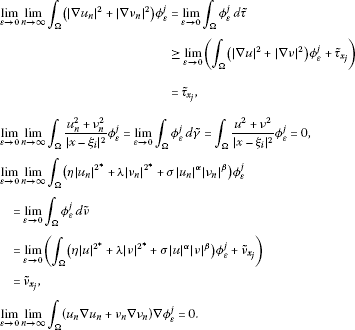Then we have

$0=\underset{\epsilon \to 0}{lim}\underset{n\to \mathrm{\infty }}{lim}〈{J}_{\lambda }^{\prime }\left({u}_{n},{\nu }_{n}\right),\left({u}_{n}{\varphi }_{\epsilon }^{j},{\nu }_{n}{\varphi }_{\epsilon }^{j}\right)〉\ge {\stackrel{˜}{\tau }}_{{x}_{j}}-{\stackrel{˜}{\nu }}_{{x}_{j}}.$

By the Sobolev inequality, ${S}_{0}{\stackrel{˜}{\nu }}_{{x}_{j}}^{\frac{2}{{2}^{\ast }}}\le {\stackrel{˜}{\tau }}_{{x}_{j}}$; and then we deduce that ${\stackrel{˜}{\nu }}_{{x}_{j}}=0$ or ${\stackrel{˜}{\nu }}_{{x}_{j}}\ge {{S}_{0}}^{\frac{N}{2}}$, which implies that $\mathcal{J}$ is finite.

Now, we consider the possibility of concentration at points ${\xi }_{i}$ ($1\le i\le k$), for $\epsilon >0$ small enough that ${x}_{j}\notin {B}_{\epsilon }\left({\xi }_{i}\right)$ for all $j\in \mathcal{J}$ and ${B}_{\epsilon }\left({\xi }_{i}\right)\cap {B}_{\epsilon }\left({\xi }_{j}\right)=\mathrm{\varnothing }$ for $i\ne j$ and $1\le i$, $j\le k$. Let ${\phi }_{\epsilon }^{i}$ be a smooth cut-off function centered at ${\xi }_{i}$ such that $0\le {\phi }_{\epsilon }^{i}\le 1$, ${\phi }_{\epsilon }^{i}=1$ for $|x-{\xi }_{i}|\ge \epsilon$ and $|\mathrm{\nabla }{\phi }_{\epsilon }^{i}|\le \frac{4}{\epsilon }$. Then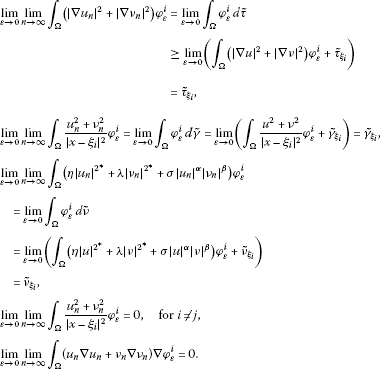Thus, we have

$0=\underset{\epsilon \to 0}{lim}\underset{n\to \mathrm{\infty }}{lim}〈{J}_{\lambda }^{\prime }\left({u}_{n},{\nu }_{n}\right),\left({u}_{n}{\phi }_{\epsilon }^{i},{\nu }_{n}{\phi }_{\epsilon }^{i}\right)〉\ge {\stackrel{˜}{\tau }}_{{\xi }_{i}}-{\mu }_{i}{\stackrel{˜}{\gamma }}_{{\xi }_{i}}-{\stackrel{˜}{\nu }}_{{\xi }_{i}}.$
(4.2)

From (4.1) and (4.2) we derive that ${S}_{{\mu }_{i}}{{\stackrel{˜}{\nu }}_{{\xi }_{i}}}^{\frac{2}{{2}^{\ast }}}\le {\stackrel{˜}{\nu }}_{{\xi }_{i}}$, $1\le i\le k$, and then either ${\stackrel{˜}{\nu }}_{{\xi }_{i}}=0$ or ${\stackrel{˜}{\nu }}_{{\xi }_{i}}\ge {S}_{{\mu }_{i}}^{\frac{N}{2}}$. On the other hand, from the above arguments, we conclude that

$\begin{array}{rcl}c& =& \underset{n\to \mathrm{\infty }}{lim}\left(J\left({u}_{n},{\nu }_{n}\right)-\frac{1}{2}〈{J}^{\prime }\left({u}_{n},{\nu }_{n}\right),\left({u}_{n},{\nu }_{n}\right)〉\right)\\ =& \frac{1}{N}\underset{n\to \mathrm{\infty }}{lim}{\int }_{\mathrm{\Omega }}\left(\eta |{u}_{n}{|}^{{2}^{\ast }}+\lambda |{\nu }_{n}{|}^{{2}^{\ast }}+\sigma |{u}_{n}{|}^{\alpha }|{\nu }_{n}{|}^{\beta }\right)\phantom{\rule{0.2em}{0ex}}dx\\ =& \frac{1}{N}\left({\int }_{\mathrm{\Omega }}\left(\eta |u{|}^{{2}^{\ast }}+\lambda |\nu {|}^{{2}^{\ast }}+\sigma |u{|}^{\alpha }|\nu {|}^{\beta }\right)\phantom{\rule{0.2em}{0ex}}dx+\sum _{j\in \mathcal{J}}{\stackrel{˜}{\nu }}_{{x}_{j}}+\sum _{i=1}^{k}{\stackrel{˜}{\nu }}_{{\xi }_{i}}\right)\\ =& \frac{1}{N}\left(\sum _{j\in \mathcal{J}}{\stackrel{˜}{\nu }}_{{x}_{j}}+\sum _{i=1}^{k}{\stackrel{˜}{\nu }}_{{\xi }_{i}}\right)+J\left(u,\nu \right).\end{array}$

If ${\stackrel{˜}{\nu }}_{{\xi }_{i}}={\stackrel{˜}{\nu }}_{{x}_{j}}=0$ for all $i\in \left\{1,\dots ,k\right\}$ and $j\in \mathcal{J}$, then $c=0$, which contradicts the assumption that $c>0$. On the other hand, if there exists an $i\in \left\{1,\dots ,k\right\}$ such that ${\stackrel{˜}{\nu }}_{{\xi }_{i}}\ne 0$ or there exists a $j\in \mathcal{J}$ with ${\stackrel{˜}{\nu }}_{{x}_{j}}\ne 0$, then we infer that

$c\ge \frac{1}{N}min\left\{{\left({S}_{\eta ,\lambda ,\sigma }\left(0\right)\right)}^{N/2},{\left({S}_{\eta ,\lambda ,\sigma }\left({\mu }_{1}\right)\right)}^{N/2},\dots ,{\left({S}_{\eta ,\lambda ,\sigma }\left({\mu }_{k}\right)\right)}^{N/2}\right\}=\frac{1}{N}{\left({S}_{\eta ,\lambda ,\sigma }\left({\mu }_{k}\right)\right)}^{N/2},$

which contradicts our assumptions. Hence, $\left({u}_{n},{\nu }_{n}\right)\to \left(u,\nu \right)$, as $n\to \mathrm{\infty }$ in $H×H$. □

First, under the assumptions (${\mathcal{H}}_{1}$), (${\mathcal{H}}_{2}$), we have the following notations:

$\begin{array}{c}{f}_{\eta ,\lambda ,\sigma }\left(\tau \right)=\frac{\left(1+{\tau }^{2}\right){S}_{{\mu }_{k}}}{{\left(\eta +\sigma {\tau }^{\beta }+\lambda {\tau }^{{2}^{\ast }}\right)}^{\frac{2}{{2}^{\ast }}}},\phantom{\rule{1em}{0ex}}\tau >0;\hfill \\ {f}_{\eta ,\lambda ,\sigma }\left({\tau }_{min}\right):=\underset{\tau >0}{min}{f}_{\eta ,\lambda ,\sigma }\left(\tau \right)>0,\phantom{\rule{1em}{0ex}}\sigma >0,\hfill \end{array}$

where ${\tau }_{min}>0$ is a minimal point of ${f}_{\eta ,\lambda ,\sigma }\left(\tau \right)$, and therefore a root of the equation

$\alpha \sigma {\tau }^{\beta }-\sigma \beta {\tau }^{\beta -2}-{2}^{\ast }\lambda {\tau }^{{2}^{\ast }-2}+{2}^{\ast }\eta =0,\phantom{\rule{1em}{0ex}}\tau >0.$

Lemma 4.2 Suppose that (${\mathcal{H}}_{1}$) holds. Then we have

1. (i)

${S}_{\eta ,\lambda ,\sigma }\left(\mu \right)={f}_{\eta ,\lambda ,\sigma }\left({\tau }_{min}\right)$

2. (ii)

${S}_{\eta ,\lambda ,\sigma }\left(\mu \right)$ has the minimizers $\left({V}_{\mu ,\epsilon }^{\xi }\left(x\right),{\tau }_{min}{V}_{\mu ,\epsilon }^{\xi }\left(x\right)\right)$, $\mathrm{\forall }\epsilon >0$, where ${V}_{\mu ,\epsilon }^{\xi }\left(x\right)$ are the extremal functions of ${S}_{\eta ,\lambda ,\sigma }\left(\mu \right)$ defined as in (2.2).

Proof The argument is similar to that of . □

Lemma 4.3 Under the assumptions of (${\mathcal{H}}_{1}$), we have

$supJ\left(t{u}_{\epsilon ,{\mu }_{k}},t\left({\tau }_{min}{u}_{\epsilon ,{\mu }_{k}}\right)\right)<{c}^{\ast }=\frac{1}{N}{\left({S}_{\eta ,\lambda ,\sigma }\left({\mu }_{k}\right)\right)}^{N/2}.$

Proof Suppose (${\mathcal{H}}_{1}$) holds. Define the function

$g\left(t\right):=J\left(t{u}_{\epsilon ,{\mu }_{k}},t\left({\tau }_{min}{u}_{\epsilon ,{\mu }_{k}}\right)\right),\phantom{\rule{1em}{0ex}}t\ge 0.$

Note that ${lim}_{t\to +\mathrm{\infty }}g\left(t\right)=-\mathrm{\infty }$ and $g\left(t\right)>0$ as t is close to 0. Thus, ${sup}_{t\ge 0}g\left(t\right)$ is attained at some finite ${t}_{\epsilon }>0$ with ${g}^{\prime }\left({t}_{\epsilon }\right)=0$. Furthermore, ${c}^{\prime }<{t}_{\epsilon }<{c}^{″}$, where ${c}^{\prime }$ and ${c}^{″}$ are the positive constants independent of ε. By using (1.2), we have

$\begin{array}{rcl}g\left(t\right)& \le & \frac{{t}^{2}}{2}\left(1+{\tau }_{min}^{2}\right)\left({\int }_{\mathrm{\Omega }}\left(|\mathrm{\nabla }{u}_{\epsilon ,{\mu }_{k}}{|}^{2}-{\mu }_{k}\frac{{u}_{\epsilon ,{\mu }_{k}}^{2}}{|x-{\xi }_{i}{|}^{2}}-{\lambda }_{1}{u}_{\epsilon ,{\mu }_{k}}^{2}\right)\phantom{\rule{0.2em}{0ex}}dx\right)\\ -\frac{{t}^{{2}^{\ast }}}{{2}^{\ast }}\left(\sigma {\tau }_{min}^{\beta }+\eta +\lambda {\tau }_{min}^{{2}^{\ast }}\right){\int }_{\mathrm{\Omega }}|{u}_{\epsilon ,{\mu }_{k}}{|}^{{2}^{\ast }}\phantom{\rule{0.2em}{0ex}}dx.\end{array}$

Note that

$max\left(\frac{{t}^{2}}{2}{B}_{1}-\frac{{t}^{{2}^{\ast }}}{{2}^{\ast }}{B}_{2}\right)=\frac{1}{N}{\left({B}_{1}{B}_{2}^{-2/{2}^{\ast }}\right)}^{N/2},\phantom{\rule{1em}{0ex}}{B}_{1}>0,{B}_{2}>0,$
(4.3)

and $0\le \mu \le \overline{\mu }-1$ and so $2<2\sqrt{\overline{\mu }-\mu }$.

From (4.3), Lemma 4.2 and Lemma 4.3, it follows that

$\begin{array}{rcl}g\left({t}_{\epsilon }\right)& \le & \frac{1}{N}{\left(\frac{\left(1+{\tau }_{min}^{2}\right){\int }_{\mathrm{\Omega }}\left(|\mathrm{\nabla }{u}_{\epsilon ,{\mu }_{k}}{|}^{2}-{\mu }_{k}\frac{{u}_{\epsilon ,{\mu }_{k}}^{2}}{|x-{\xi }_{k}{|}^{2}}-{\lambda }_{1}{u}_{\epsilon ,{\mu }_{k}}^{2}\right)\phantom{\rule{0.2em}{0ex}}dx}{{\left(\left(\sigma {\tau }_{min}^{\beta }+\eta +\lambda {\tau }_{min}^{{2}^{\ast }}\right){\int }_{\mathrm{\Omega }}|{u}_{\epsilon ,{\mu }_{k}}{|}^{{2}^{\ast }}\phantom{\rule{0.2em}{0ex}}dx\right)}^{2/{2}^{\ast }}}\right)}^{N/2}\\ \le & \frac{1}{N}\left(\frac{f\left({\tau }_{min}\right)}{S\left({\mu }_{k}\right)}×\frac{{\left(S\left({\mu }_{k}\right)\right)}^{N/2}+O\left({\epsilon }^{2\sqrt{\overline{\mu }-\mu }}\right)-C{\epsilon }^{2}}{{\left(S\left({\mu }_{k}\right)\right)}^{\left(N-2\right)/2}+O\left({\epsilon }^{2\sqrt{\overline{\mu }-\mu }}\right)}\right)\\ \le & \frac{1}{N}{\left(f\left({\tau }_{min}\right)\right)}^{N/2}+O\left({\epsilon }^{2\sqrt{\overline{\mu }-\mu }}\right)-C{\epsilon }^{2}\\ <& \frac{1}{N}{\left({S}_{\eta ,\lambda ,\sigma }\left({\mu }_{k}\right)\right)}^{N/2}={c}^{\ast },\end{array}$

so $g\left({t}_{\epsilon }\right)<{c}^{\ast }$. Hence, $g\left({t}_{\epsilon }\right)<{c}^{\ast }$, $\mathrm{\forall }t\ge 0$ and

(4.4)

□

Proof of Theorem 1.3 Set $c:={inf}_{h\in \mathrm{\Gamma }}{max}_{t\in \left[0,1\right]}J\left(h\left(t\right)\right)$, where

$\mathrm{\Gamma }=\left\{h\in C\left(\left[0,1\right],H×H\right)|h\left(0\right)=\left(0,0\right),J\left(h\left(1\right)\right)<0\right\}.$

Suppose that (${\mathcal{H}}_{1}$) holds. For all $\left(u,\nu \right)\in H×H\mathrm{\setminus }\left\{\left(0,0\right)\right\}$, from the Young and Hardy-Sobolev inequalities, it follows that

$\begin{array}{rcl}J\left(u,\nu \right)& \ge & C\left({\parallel u\parallel }^{2}+{\parallel \nu \parallel }^{2}\right)-C\left({\parallel u\parallel }^{{2}^{\ast }}+{\parallel \nu \parallel }^{{2}^{\ast }}\right)\\ \ge & C{\parallel \left(u,\nu \right)\parallel }^{2}-C{\parallel \left(u,\nu \right)\parallel }^{{2}^{\ast }},\end{array}$

and there exists a constant $\rho >0$ small such that

$b:=\underset{\parallel \left(u,\nu \right)\parallel =\rho }{inf}J\left(u,\nu \right)>0=J\left(0,0\right).$

Since $J\left(tu,t\nu \right)\to -\mathrm{\infty }$ as $t\to \mathrm{\infty }$, there exists ${t}_{0}>0$ such that $\parallel \left({t}_{0}u,{t}_{0}\nu \right)\parallel >\rho$ and $J\left({t}_{0}u,{t}_{0}\nu \right)<0$. By the mountain-pass theorem , there exists a sequence $\left\{\left({u}_{n},{\nu }_{n}\right)\right\}\subset H×H$ such that $J\left({u}_{n},{\nu }_{n}\right)\to c$ and ${J}^{\prime }\left({u}_{n},{\nu }_{n}\right)\to 0$, as $n\to \mathrm{\infty }$.

From Lemma 4.2 it follows that

$\begin{array}{rcl}0

By Lemma 4.1 there exists a subsequence of $\left\{\left({u}_{n},{\nu }_{n}\right)\right\}$, still denoted by $\left\{\left({u}_{n},{\nu }_{n}\right)\right\}$, such that $\left({u}_{n},{\nu }_{n}\right)\to \left(u,\nu \right)$ strongly in $H×H$. Thus, we get a critical point $\left(u,\nu \right)$ of J satisfying (1.1), and c is a critical value. Set ${u}^{+}=max\left\{u,0\right\}$.

Replacing respectively u, ν with ${u}^{+}$ and ${\nu }^{+}$ in terms of the right-hand side of (1.1) and repeating the above process, we can get a nonnegative nontrivial solution $\left(u,\nu \right)$ of (1.1). If $u\equiv 0$, we get $\nu \equiv 0$ by (1.1) and the assumption ${a}_{2}>0$. Similarly, if $\nu \equiv 0$, we also have $u\equiv 0$. There, $u,\nu \not\equiv 0$. From the maximum principle, it follows that $u,\nu >0$ in Ω. □

## References

1. Cao D, Han P: Solutions to critical elliptic equations with multi-singular inverse square potentials. J. Differ. Equ. 2006, 224: 332-372. 10.1016/j.jde.2005.07.010

2. Hsu TS: Multiple positive solutions for semilinear elliptic equations involving multi-singular inverse square potentials and concave-convex nonlinearities. Nonlinear Anal. 2011, 74: 3703-3715. 10.1016/j.na.2011.03.016

3. Kang D: On the weighted elliptic problems involving multi-singular potentials and multi-critical exponents. Acta Math. Sin. Engl. Ser. 2009, 25: 435-444. 10.1007/s10114-008-6450-7

4. Abdellaoui B, Felli V, Peral I:Some remarks on systems of elliptic equations doubly critical in the whole ${\mathbb{R}}^{N}$. Calc. Var. Partial Differ. Equ. 2009, 34: 97-137. 10.1007/s00526-008-0177-2

5. Bouchekif M, Nasri Y: On a singular elliptic system at resonance. Ann. Mat. Pura Appl. 2010, 189: 227-240. 10.1007/s10231-009-0106-9

6. Huang Y, Kang D: On the singular elliptic systems involving multiple critical Sobolev exponents. Nonlinear Anal. 2011, 74: 400-412. 10.1016/j.na.2010.08.051

7. Kang D: Semilinear systems involving multiple critical Hardy-Sobolev exponents and three singular points. Appl. Math. 2011, 218: 4514-4522.

8. Kang D, Peng S: Existence and asymptotic properties of solutions to elliptic systems involving multiple critical exponents. Sci. China Math. 2011, 54(2):243-256. 10.1007/s11425-010-4131-3

9. Kang D, Huang Y, Liu S: Asymptotic estimates on the extremal functions of a quasi-linear elliptic problem. J. South-Central Univ. Natl. Nat. Sci. Ed. 2008, 27(3):91-95.

10. Caffarelli L, Kohn R, Nirenberg L: First order interpolation inequality with weights. Compos. Math. 1984, 53: 259-275.

11. Cai, M, Kang, D: Concentration-compactness principles for the systems of critical elliptic equations. Acta Math. Sci. Ser. B Engl. Ed. (to appear)

12. Lions PL: The concentration-compactness principle in the calculus of variations: the limit case I. Rev. Mat. Iberoam. 1985, 1: 45-121.

13. Lions PL: The concentration-compactness principle in the calculus of variations: the limit case II. Rev. Mat. Iberoam. 1985, 1: 145-201.

14. Ambrosetti A, Rabinowitz PH: Dual variational methods in critical point theory and applications. J. Funct. Anal. 1973, 14: 349-381. 10.1016/0022-1236(73)90051-7

## Author information

Authors

### Competing interests

The authors declare that they have no competing interests.

### Authors’ contributions

Each of the authors, SK, MF and OKK contributed to each part of this work equally and read and approved the final version of the manuscript.

## Rights and permissions

Reprints and Permissions

Khademloo, S., Farzinejad, M. & Khazaee kohpar, O. Existence result for semilinear elliptic systems involving critical exponents. Bound Value Probl 2012, 119 (2012). https://doi.org/10.1186/1687-2770-2012-119

• Accepted:

• Published:

• DOI: https://doi.org/10.1186/1687-2770-2012-119

### Keywords

• semilinear elliptic system
• nontrivial solution
• critical exponent
• variational method